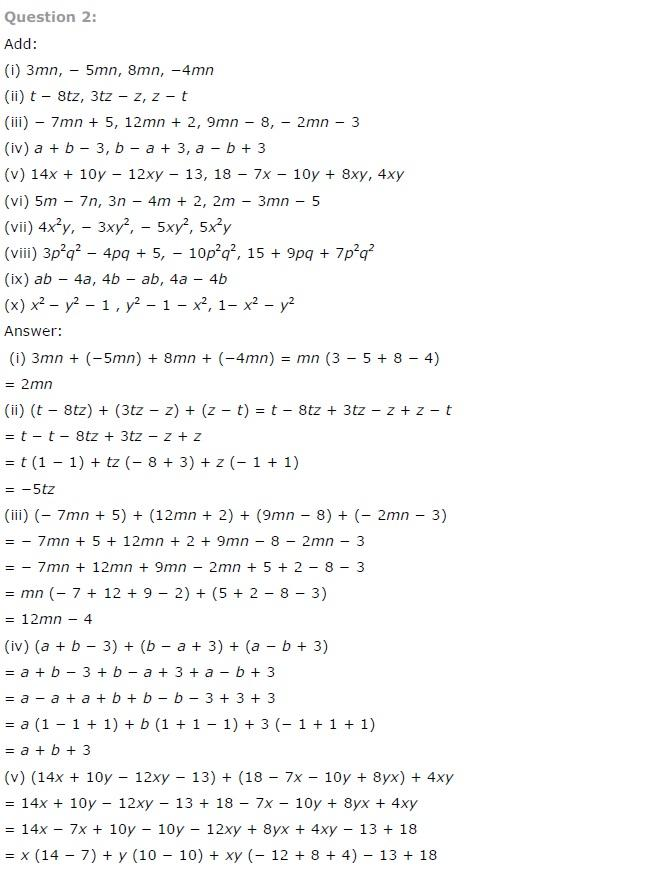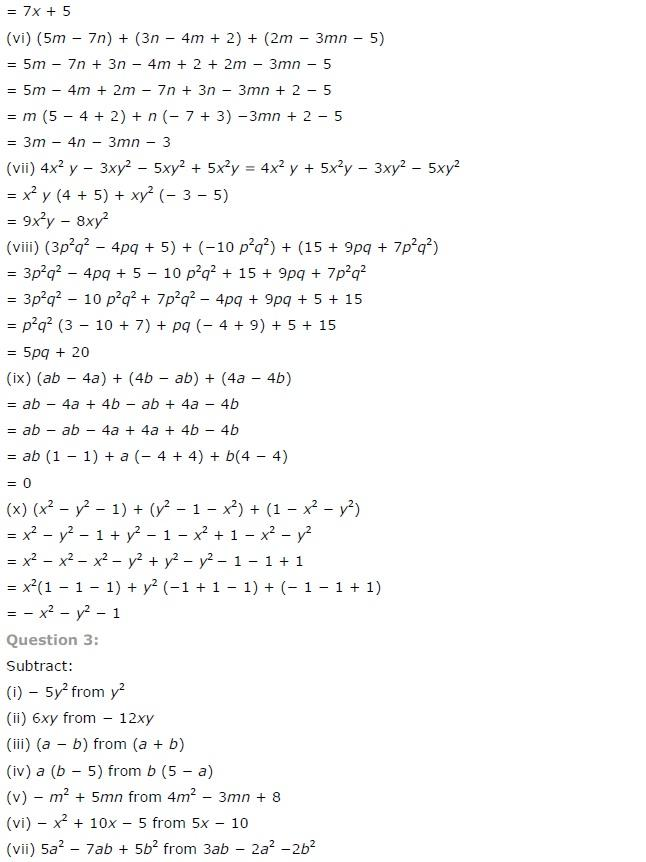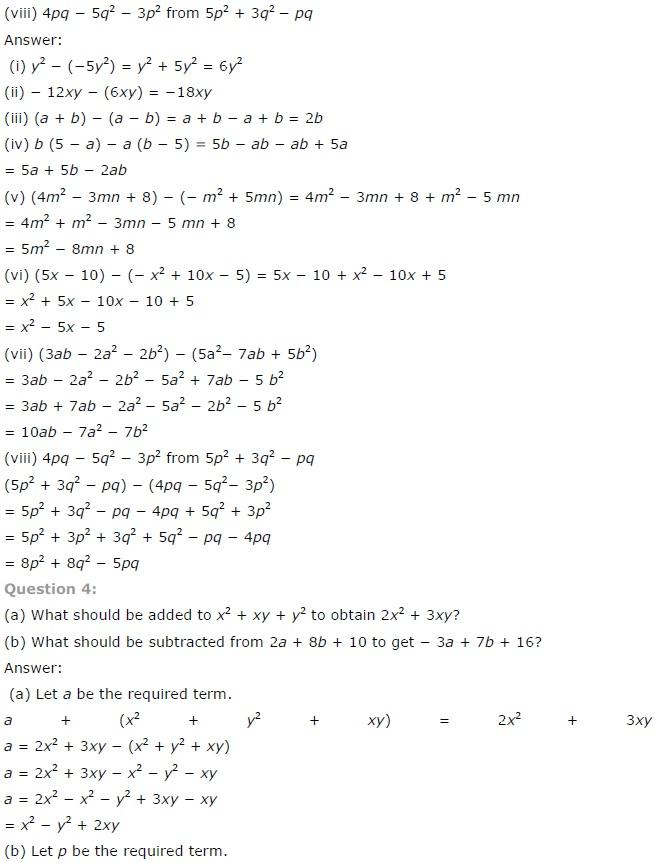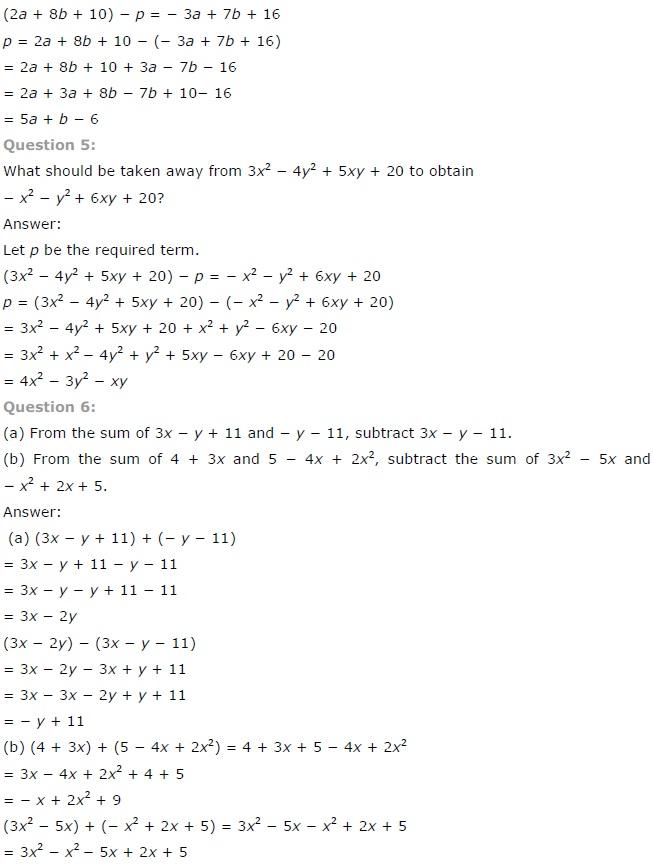# NCERT Solutions class 7 Maths Chapter-12 Exercise 12.2

## NCERT Solutions class 7 Maths Chapter-12 Algebraic expressions

### Algebraic expressions  Exercise-12.2

NCERT Solutions Class-7 Maths chapter-12 Algebraic expressions  Exercise-12.2 is prepared by academic team of pw all the questions of NCERT text book are solved step by step with proper and detail solutions explaining each and every questions . For More and additional questions of CBSE class 7 maths you can go to class 7 maths sections. NCERT class 7 Maths Solutions is the best way to enhanced your mathematics skill. And pw practice worksheet & question bank will help you a lot .

### Solutions of Chapter Algebraic expressions  Exercise-12.2

Question 1:

Simplify combining like terms:

(i) 21b - 32 + 7b - 20b

(ii)-z2 + 13z2 - 5z + 7z3 -15z

(iii) p -(p - q) - q - (q - p)

(iv) 3a - 2b - ab -(a - b + ab) + 3ab + b -a

(v) 5x2y -5x2 + 3yx2 -3y2 + x2 - y2 + 8xy2 -3y2

(vi) (3y2 + 5y -4) - (8y - y2 -4)

The parts of the given questions are solved as follows:

(i) It is given in the question that,

We have to simplify the expression by combining like terms:

21b - 32 + 7b - 20b

= 21b – 20d +7b - 32

= (21 – 20 + 7)b – 32

= 8b – 32

(ii) It is given in the question that,

We have to simplify the expression by combining like terms:

-z2 + 13z2 - 5z + 7z3 -15z

= - z2 + 13z2 + 7z3 – 5z – 15z

= z2(- 1 + 13) + 7z3 – z(5+15)

= 12z2 + 7z3 – 20z

(iii) It is given in the question that,

We have to simplify the expression by combining like terms:

p -(p - q) - q - (q - p)

= p – p + q – q – q +p

= p – p + p + q – q – q

= p – q

(iv) It is given in the question that,

We have to simplify the expression by combining like terms:

3a - 2b - ab -(a - b + ab) + 3ab + b -a

= 3a – 2b – ab – a + b – ab + 3ab + b – a

= 3a – a – a – 2b + b + b – ab – ab + 3ab

= (3 – 1 – 1)a–b(2 – 1 - 1) -ab(1 + 1 – 3)

= a + ab

(v) It is given in the question that,

We have to simplify the expression by combining like terms:

5x2y -5x2 + 3yx2 -3y2 + x2 - y2 + 8xy2 -3y2

= 5x2y + 3x2y – 5x2 + x2 – 3y2 –y2 – 3y2 + 8xy2

= (5 + 3)x2y + (– 5 + 1)x2 + (– 3 – 1 – 1)y2 + 8xy2

= 8x2y – 4x2 – 5y2 + 8xy2

(vi) It is given in the question that,

We have to simplify the expression by combining like terms:

(3y2 + 5y -4) - (8y - y2 -4)

= 3y2+ 5y – 4 – 8y + y2 + 4

= 3y2 + y2 + 5y – 8y – 4 + 4

= 4y2 – 3yNCERT CLASS 7 MATHEMATICS SOLUTIONS